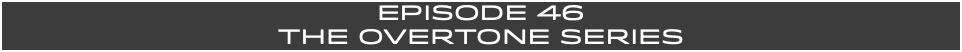HOSTS- Jeremy Burns, Matthew Scott Phillips

TYPE- Special Topics

DURATION- 67:57

BUMPER MUSIC- "The Cold Comfort of Toys" (Matthew Scott Phillips)

ANNOUNCER- Mike Cunliffe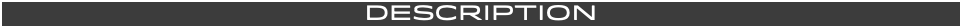The overtone series is alive and well in every note you hear. Within the vibrations of a tone, or a note, there are other smaller vibrations that are occurring. We will start with the fundamental and look deeper into the overtones, or harmonics, that occur and at which order they appear. We will also discuss timbre and other things that are influenced by and, likewise, influence certain overtones.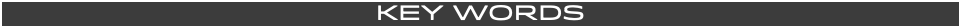TONE- A musical sound that rings at a certain frequency. The frequency determines what pitch the tone will sound as.

FREQUENCY- The number of vibrations per second. Frequencies are measured in hertz (Hz). For example, the note A 440Hz vibrates at a frequency of 440 time per second.

HERTZ- The unit of measurement that is determined by the amount of times something vibrates in a second.

FUNDAMENTAL- This is the most prominent tone that rings out. The tone that rings as the fundamental is often the note that is played. For example, if you play the A note above middle C, than that note (A 440) is considered the fundamental. This is usually the most audible tone.

OVERTONE- This is a frequency or tone that is added to another tone. This is also referred to as a "harmonic". The FUNDAMENTAL is considered the "1st harmonic". The "2nd harmonic" is known as the "1st overtone".

NODE- This is a point of minimal amplitude, where the vibration is divided into smaller vibrations.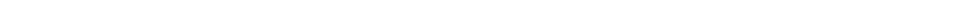THE FOLLOWING OVERTONE SERIES EXPLANATION WILL BE BASED FROM THE FUNDAMENTAL NOTE OF

A (110Hz).

OF COURSE, THIS SERIES COULD RISE FROM ANY STARTING NOTE.

THE SAME RATIOS WILL APPLY.The FUNDAMENTAL, in this case, will be the note

A (110Hz), 2 octaves below middle C. This graphic shows how the open low A string, on a guitar, will vibrate when you play it. The FUNDAMENTAL is known as the 1st HARMONIC.While this string is vibrating, there are sub divisions of the string that are also vibrating. These

"sub vibrations" are known as OVERTONES.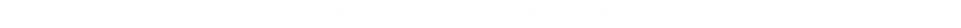The 1st OVERTONE, in this case, will be the note

A (220 Hz), an octave above the FUNDAMENTAL. This graphic shows how the open low A string, on a guitar, is also vibrating at a quieter volume at twice the frequency. This 1st OVERTONE is ringing at a 2:1 ratio. There are 2 vibrations for each 1 of the FUNDAMENTAL. The point at the middle of the string, from where this overtone originates and vibrates outward, is referred to as a NODE.The 2nd OVERTONE, of this series, will be the note E (330 Hz), an octave + a perfect 5th above the FUNDAMENTAL. The ratio for this overtone is a 3:1 ratio, as depicted below.The 3rd OVERTONE will be the note A (440 Hz), 2 octaves above the FUNDAMENTAL. The ratio for this overtone is a 4:1 ratio, as depicted below.The 4th OVERTONE will be the note C# (550 Hz), 2 octaves + a major 3rd above the FUNDAMENTAL.

The ratio for this overtone is a 5:1 ratio.The 5th OVERTONE will be the note E (660 Hz),

2 octaves + a perfect 5th above the FUNDAMENTAL.

The ratio for this overtone is a 6:1 ratio.The 6th OVERTONE will be the note G (770 Hz),

2 octaves + a minor 7th above the FUNDAMENTAL.

The ratio for this overtone is a 7:1 ratio.Here is a look at all of these together.This pattern doesn't merely repeat. As we move up in the series, other notes (such as the minor 3rd, the 9th and the 11th) are also present. They are barely audible, if at all. But they are, nevertheless, all present within the FUNDAMENTAL tone.

The previous examples involved perfect ratios.

This is because of the use of the "JUST TUNING" or "PYTHAGOREAN TUNING" system. Below, is the same diagram with the use of the "EQUAL TEMPERAMENT" system. Notice that instead of nice "round" numbers, we often get decimal points for some of these overtones (2nd, 4th and 6th).-When you think you are hearing a single note, you are actually hearing many other notes at quieter levels. These are known as "OVERTONES" or "HARMONICS".

-Music in the Middle Ages mainly featured notes based on the 1st couple of overtones (the octave and the 5th). Notes based on other overtones became more common. The major 3rd (4th overtone) became prevalent in the Renaissance and the minor 7th found its way into the Baroque era. This gave rise to the every popular V7 chord.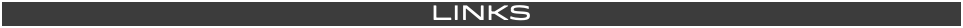"9. The Overtone Series and Timbre"

by Walk That Bass

"Why It's Impossible to Tune a Piano"

by Minute Physics

MUSIC STUDENT 101